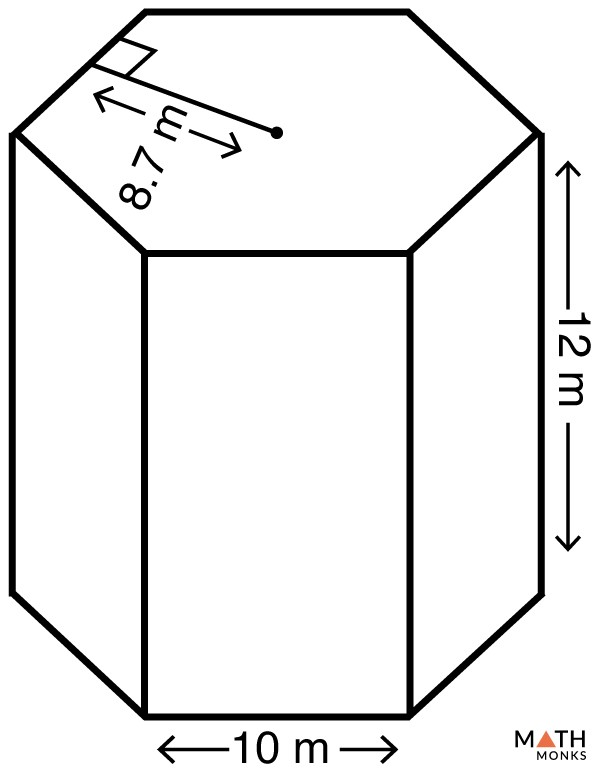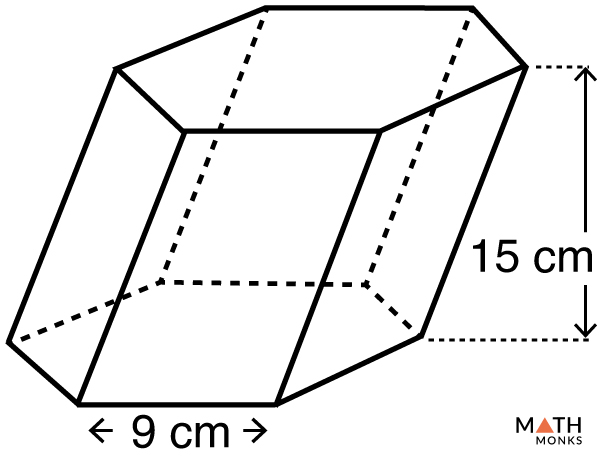# Volume of a Hexagonal Prism

The volume of a hexagonal prism is the space it occupies in the three-dimensional plane. It is measured in cubic units such as m3, cm3, mm3, ft3.

## Formula

The formula is given below:

Let us solve some examples to understand the concept better.

## Solved ExamplesFind the volume of a hexagonal prism with a base edge of 10 m, apothem of 8.7 m and a height of 12 m.

Solution:

As we know,
Volume (V) = 3abh, here a = 8.7 m, b = 10 m, h = 12 m
V = 3 × 10 × 8.7 × 12
= 3132 cm3

Finding the volume of a hexagonal prism when BASE EDGE and HEIGHT are knownFind the volume of a hexagonal prism with a base edge of 6.5 cm and a height of 10.8 cm.

Solution:

Here we will use an alternate formula.
Volume (V) = ${\dfrac{3\sqrt{3}}{2}\times a^{2}\times h}$ , here b = 6.5 cm, h = 10.8 cm
V = ${\dfrac{3\sqrt{3}}{2}\times 6.5^{2}\times 10.8}$
≈ 1185.502 cm3

Finding the volume of an oblique hexagonal prism when BASE EDGE and HEIGHT are knownFind the volume of a oblique hexagonal prism given in the figure.

Solution:

As we know,
The volume of an oblique hexagonal prism is same as the volume of a right hexagonal prism having the height ‘h’
Volume (V) = ${\dfrac{3\sqrt{3}}{2}\times a^{2}\times h}$ , here a = 9 cm, h = 15 cm
V = ${\dfrac{3\sqrt{3}}{2}\times 9^{2}\times 15}$
= 3156.67 cm3

• More Resources: